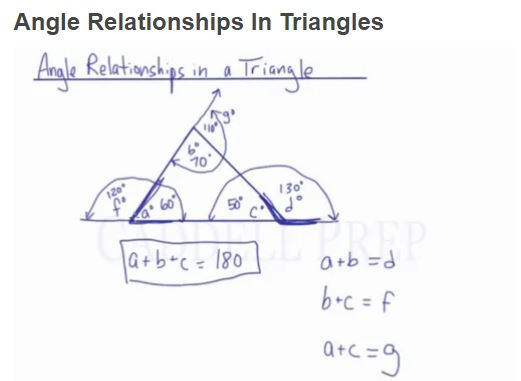In this video, we are going to look at the angle relationships in a triangle.

Let’s label the angles$a\textdegree$,$b\textdegree$, and$c\textdegree$. The most common rule for angles in a triangle is:$a\textdegree + b\textdegree + c\textdegree = 180\textdegree$

If we extend one side (past angle c as shown in the video) and label it$d\textdegree$, then there is another rule, which works for all exterior angles.$a\textdegree+b\textdegree=d\textdegree$

For example:
If$a\textdegree=60\textdegree$ and$b\textdegree=70\textdegree$, then$c\textdegree$ must be$50\textdegree$. Since$c=50\textdegree$, then$d$ must be$130\textdegree$ because they lie on a straight line. Therefore,$a\textdegree+b\textdegree=d\textdegree$.## Video-Lesson Transcript

In this lesson, we’ll cover angle relationships in a triangle.

A triangle has three angles.

Let’s label them as$a^{\circ}$,$b^{\circ}$, and$c^{\circ}$.

If we add this all up:$a^{\circ} + b^{\circ} + c^{\circ} = 180$

If we extend the horizontal line of the triangle going to the right, we will form a new angle. Let’s call this angle$d^{\circ}$.$a^{\circ} + b^{\circ} = d^{\circ}$

Let’s give values to this:$a^{\circ} = 60^{\circ}$$b^{\circ} = 70^{\circ}$$c^{\circ} = 50^{\circ}$

Since$c^{\circ} = 50^{\circ}$,$d^{\circ}$ should be$130^{\circ}$ because$c$ and$d$ forms$180^{\circ}$

This is also because:$a + b + c = 180$
and$c + d = 180$$c$ remains the same in both situations so$a + b = d$.

This is true for any of these.

If you will extend the horizontal line of the triangle going to the left, let’s label this$f^{\circ}$.

Then$b + c = f$

So, if$a = 60^{\circ}$ and$a + f = 180$, therefore$f = 120^{\circ}$.

In this case, we proved that$b + c = f$.

Now, let’s extend the line with angle$b$ and call it angle$g^{\circ}$.

Since$b = 70^{\circ}$, the angle beside it is$110^{\circ}$.

Therefore,$a + c = g$

The sum of all the interior angles is equal to$180$.

And the exterior angles is equal to the sum of the other two interior angles.